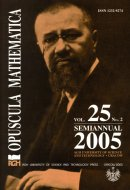Opuscula Math. 25, no. 2 (2005), 269-274

Opuscula Mathematica

# 2-biplacement without fixed points of (p,q)-bipartite graphs

Beata Orchel

Abstract. In this paper we consider $$2$$-biplacement without fixed points of paths and $$(p,q)$$-bipartite graphs of small size. We give all $$(p,q)$$-bipartite graphs $$G$$ of size $$q$$ for which the set $$\mathcal{S}^{*}(G)$$ of all $$2$$-biplacements of $$G$$ without fixed points is empty.

Keywords: bipartite graph, packing, embedding.

Mathematics Subject Classification: 05C35.

Full text (pdf)

• Beata Orchel
• AGH University of Science and Technology, Faculty of Applied Mathematics, al. Mickiewicza 30, 30-059 Kraków, Poland